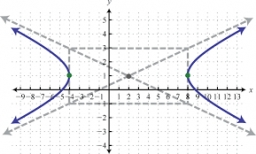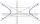# Hyperbola equation

Find the hyperbola equation with the center of S [0; 0], passing through the points:
A [5; 3] B [8; -10]

Result

f = (Correct answer is: f = 7 * x^2 - 3y^2 = 148)#### Solution:We would be pleased if you find an error in the word problem, spelling mistakes, or inaccuracies and send it to us. Thank you!Tips to related online calculators
Looking for help with calculating roots of a quadratic equation?
Do you have a linear equation or system of equations and looking for its solution? Or do you have quadratic equation?

#### You need to know the following knowledge to solve this word math problem:

We encourage you to watch this tutorial video on this math problem:

## Next similar math problems:

• Equation - inverseSolve for x: 7: x = 14: 1000
• HyperbolaFind the equation of hyperbola that passes through the point M [30; 24] and has focal points at F1 [0; 4 sqrt 6], F2 [0; -4 sqrt 6].